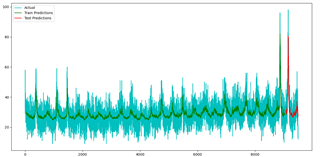This discussion has been locked.
You can no longer post new replies to this discussion. If you have a question you can start a new discussion

# Improving an LSTM model fit to a datasets noise

I am a data analyst trying to improve my knowledge of machine learning.

I've completed a model for a time-series dataset, where each point is 1 day apart, with no gaps. The specific model type that I have attempted is a multi-layered auto-regression testing tool bi-directional LSTM using tensorflow's keras, see model-specific code below:

```model = keras.Sequential()
units = 128,
input_shape = (X_train.shape, X_train.shape),
return_sequences=True)))
units = 64,
input_shape = (X_train.shape, X_train.shape),
return_sequences=True)))
units = 32,
input_shape = (X_train.shape, X_train.shape),
return_sequences=True)))
units = 16,
input_shape = (X_train.shape, X_train.shape),
return_sequences=False)))
history = model.fit(
X_train, y_train,
epochs = 100,
batch_size = 128,
validation_split = 0.2,
shuffle = False
)
print(model.summary())```

I've been told that this is likely overkill for this specific learning task by a superior member of staff but wanted to add it for full transparency. See summary below:

``` Layer (type)                Output Shape              Param #
=================================================================
bidirectional (Bidirectiona  (None, 50, 256)          133120
l)

bidirectional_1 (Bidirectio  (None, 50, 128)          164352
nal)

bidirectional_2 (Bidirectio  (None, 50, 64)           41216
nal)

bidirectional_3 (Bidirectio  (None, 32)               10368
nal)

dense (Dense)               (None, 16)                528

dropout (Dropout)           (None, 16)                0

dense_1 (Dense)             (None, 1)                 17

=================================================================
Total params: 349,601
Trainable params: 349,601
Non-trainable params: 0
_________________________________________________________________```

The model reports the loss values (after 100 epochs, using Mean Squared Error):

loss: 0.0040 - val_loss: 0.0050 (Overfit)

With an RMSE derived with: `math.sqrt(mean_squared_error(y_train,train_predict))` and `math.sqrt(mean_squared_error(y_test,test_predict))` with `sklearn.metrics` and the built-in function `mean_squared_error` from the aforementioned package.

Train RMSE: 28.795422522129595

Test RMSE: 34.17014386085355

And for a graphical representation:To which I finally arrive at my question; how do I better fit my model to more closely representing the noise within the data, as this is what I believe to be causing the high RMSE values. I have looked into attention mechanisms, in the hopes that I might be able to highlight specific peaks and troughs within the data, but it seems that these are best used with image/text prediction oriented models. I could try training over more epochs, but the model is already slightly overfitting, so this would exasperate this particular issue further.

I understand this is a fairly open-ended question but I have best tried to "show my working" and thank you in advance.

Parents
• Hi!

The task sounds like function extrapolation.

Could you describe what inputs/outputs are used in the model? Is it some sort of floating window?